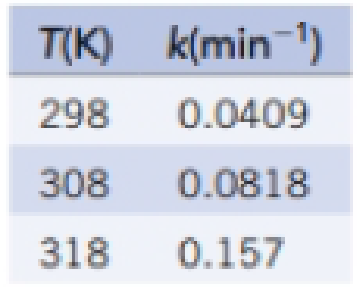# Data for the reaction [Mn(CO) 5 (CH 3 CN)] + + NC 5 H 5 → (Mn(CO) 5 (NC 5 H 5 )] + + CH 3 CN are given in the table. Calculate E a from a plot of In k versus 1/ T.### Chemistry & Chemical Reactivity

9th Edition
John C. Kotz + 3 others
Publisher: Cengage Learning
ISBN: 9781133949640

#### Solutions

Chapter
Section### Chemistry & Chemical Reactivity

9th Edition
John C. Kotz + 3 others
Publisher: Cengage Learning
ISBN: 9781133949640
Chapter 14, Problem 69GQ
Textbook Problem
53 views

## Data for the reaction[Mn(CO)5(CH3CN)]+ + NC5H5 → (Mn(CO)5(NC5H5)]+ + CH3CNare given in the table. Calculate Ea from a plot of In k versus 1/T.Interpretation Introduction

Interpretation:

The value for Ea should be determined using the plot obtained by the given set of data.

Concept introduction:

Rate law: It is generally the rate equation that consists of the reaction rate with the concentration or the pressures of the reactants and constant parameters.

Rate constant: The rate constant for a chemical reaction is the proportionality term in the chemical reaction rate law which gives the relationship between the rate and the concentration of the reactant present in the chemical reaction.

Rate order: The order of each reactant in a reaction is represented by the exponential term of the respective reactant present in the rate law and the overall order of the reaction is the sum of all the exponents of all reactants present in the chemical reaction.  The order of the reaction is directly proportional to the concentration of the reactants.

Arrhenius equation:

• Arrhenius equation is a formula that represents the temperature dependence of reaction rates
• The Arrhenius equation has to be represented as follows

k=AeEa/RTlnk=lnAeEa/RTlnk=(EaR)(1T)+lnA

• Ea represents the activation energy and it’s unit is kJ/mol
• R represents the universal gas constant and it has the value of 8.314 J/K.mol
• T represents the absolute temperature
• A represents the frequency factor or collision frequency
• e represents the base of natural logarithm
•  Arrhenius equation equation was proposed by Svante Arrhenius in 1889.

### Explanation of Solution

For the given reaction lnk and 1/T are tabulated as follows by using the given set of data.

The corresponding graph is as follows,

Findthe slope for any two values present in the graph:slope=-1.852-(-2.503)0.003145-0

### Still sussing out bartleby?

Check out a sample textbook solution.

See a sample solution

#### The Solution to Your Study Problems

Bartleby provides explanations to thousands of textbook problems written by our experts, many with advanced degrees!

Get Started

Find more solutions based on key concepts
According to a national 2010 health report, a. most people's diets lacked enough fruits, vegetables, and whole ...

Nutrition: Concepts and Controversies - Standalone book (MindTap Course List)

What are the features of a healthy high-fat diet?

Understanding Nutrition (MindTap Course List)

Explain the structure and function of the endomembrane system.

Biology: The Dynamic Science (MindTap Course List)

Provide two reasons why meiosis leads to genetic variation in diploid organisms.

Human Heredity: Principles and Issues (MindTap Course List)

Give general chemical formulas for a primary, a secondary, and a tertiary amine.

Chemistry for Today: General, Organic, and Biochemistry

In Figure P19.22, the change in internal energy of a gas that is taken from A to C along the blue path is +800 ...

Physics for Scientists and Engineers, Technology Update (No access codes included)

What is the molecular formula for 2, 2, 3-trimethylbutane?

Introductory Chemistry: An Active Learning Approach